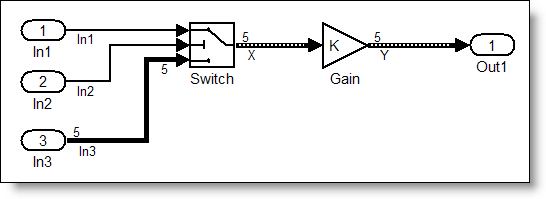# Generated Code for Variable Size Signals

Aarti recently posted about Variable Size Signals in Simulink. Han responded with this comment:

Aarti,
I would be interested in the effect on RTW generated code for variable size signals. Could you show some examples?
-Han

Here is Aarti's response.Hi Han,

Thank you for your comment. In the case of a variable size signal, the generated code allocates the maximum possible size for the input/output variables.

This allows signal sizes to vary during execution (as opposed to being hard-coded after model compilation). The generated code also propagates signal sizes through the model and accordingly assign the required dimension to signals. The algorithm code only needs to operate on the portion of memory required (thus saving execution time).

The following model uses the Switch Block to change the size of its output signal.The generated code looks like this:


/* Switch: '/Switch' incorporates:
*  Inport: '/In1'
*  Inport: '/In2'
*  Inport: '/In3'
*/
if (In2 >= 0.0) {
model_XDim = 1;
X = In1;
} else {
model_XDim = 5;
for (tmp = 0; tmp < 5; tmp++) {
X[tmp] = In3[tmp];
}
}

/* Gain: '/Gain' */
model_YDim = model_XDim;
loop_ub = model_XDim - 1;
for (tmp = 0; tmp <= loop_ub; tmp++) {
Y[tmp] = 2.0 * X[tmp];
}


In the generated code, notice how the variable model_Xdim has the lower and upper bound pre-allocated as 1 and 5, 5 being the width of the 3rd Inport block. Using these values, the loop_ub variable for the Switch block is calculated.

Thanks!

Aarti